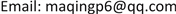DSCDynamical Systems and Control2325-677XScientific Research Publishing10.12677/DSC.2018.74035DSC-27295DSC20180400000_11967699.pdf工程技术 基于PLC的非线性水箱的预测控制研究 Research on Predictive Control of Nonlinear Water Tank Based on PLC 21宝林213141青鹏41冠甫541国电科学技术研究院有限公司，江苏 南京华北电力大学(保定)，河北 保定 国电科学技术研究院有限公司，江苏 南京 华北电力大学(保定)，河北 保定null060920180704310317© Copyright 2014 by authors and Scientific Research Publishing Inc. 2014This work is licensed under the Creative Commons Attribution International License (CC BY). http://creativecommons.org/licenses/by/4.0/

1国电科学技术研究院有限公司，江苏 南京

2华北电力大学(保定)，河北 保定Copyright © 2018 by authors and Hans Publishers Inc.1. 引言

2. 动态矩阵控制(DMC)2.1. 基本原理介绍

DMC算法包含三个部分：预测模型、滚动优化、反馈校正  。其算法流程图如图1所示。

2.2. 基于黄金分割法的有约束预测控制器设计

2.2.1. 黄金分割算法介绍

(1) 确定收敛精度 ε ；

(2) 从 [ a , b ] 区间内选择 r 1 , r 2 ( r 1 < r 2 ) 两点，然后将 [ a , b ] 分成三段；

(3) 判断 f ( r 1 ) 和 f ( r 2 ) 的大小，若 f ( r 1 ) > f ( r 2 ) ，则收敛区间则需要更新：

a = r 1

r 1 = r 2

r 2 = a + 0.618 × ( b − a )

b = r 2

r 2 = r 1

r 1 = a + 0.382 × ( b − a )

(4) 若 b − a a < ε 则停止迭代，极值点为 a + b 2 ，函数的极值为 f ( a + b 2 ) ；否则，返回步骤(3)继续迭代。

2.2.2. 基于黄金分割法的有约束预测控制器设计

min J ( k ) = ‖ w p ( k ) − y ˜ p 0 ( k ) − A 2 Δ u ( k ) ‖ Q 2 + H T R H Δ u 2 ( k )

Δ u ( k ) = arg min J ( Δ u ( k ) ) s . t . Δ u ( k + i ) ∈ [ Δ u min , Δ u max ] , Δ u min < 0 , Δ u max > 0 u ( k + i ) ∈ [ u min , u max ] , u min ≥ 0 , Δ u max > 0 Δ u ( k + i ) = γ i Δ u ( k ) , 1 ≤ i ≤ M − 1

u ( k ) = Δ u ( k ) + u ( k − 1 ) u ( k + 1 ) = γ Δ u ( k ) + Δ u ( k ) + u ( k − 1 ) u ( k + M − 1 ) = R i Δ u ( k ) + u ( k − 1 )

Δ u ( k ) ∈ { [ max ( Δ u min , u min − u ( k − 1 ) R M − 1 ) , min x ( Δ u max , u max − u ( k − 1 ) R M − 1 ) ] , γ < 1 [ max ( Δ u min γ M − 1 , u min − u ( k − 1 ) R M − 1 ) , min x ( Δ u max γ M − 1 , u max − u ( k − 1 ) R M − 1 ) ] , γ ≥ 1 }

3. MATLAB仿真实例3.1. 控制对象的辨识及参数的获取

G ( s ) = 3.7766 ( 52.676 s + 1 ) 2

3.2. DMC算法和PID算法的比较

4. 基于PLC的水箱控制试验4.1. 实验设备介绍

A3030型艾默生过程自动化实训系统通过实际流体(自来水)的流量控制和液位控制来模拟对实际对象的控制和设备管理，它充分展示了DCS分散控制功能，体现了控制系统和现场仪表间的协同运作能力以及在PID回路之外建立设备管理平台为用户带来预诊断功能的先进理念，由于上水箱是一个圆筒状模型，一个具有典型非线性特征的对象，可以模拟很多实际工业过程被控对象。

4.2. 实验流程及实例

OVATION是美国开发的新型分散控制系统，它既包含过程控制技术，又吸收了企业管理信息技术，系统在硬件上采用了速度快、容量大的设备，保证系统的高性能运行；通讯网络使用标准的以太网，可靠性高、速度快、开放性高，提升其多任务、多数据的工作能力。

5. 结论